# Launch Time: The Physics of Catapult Projectile Motion

 Areas of Science Physics Difficulty Time Required Very Short (≤ 1 day) Prerequisites This project requires a basic understanding of algebra, trigonometry (sine and cosine functions), and physics (kinematics—two-dimensional projectile motion), or the willingness to learn about these subjects on your own. Material Availability This project requires access to a video camera (not included in the cost estimate) and the purchase of a kit. A Ping Pong Catapult Kit is available from our partner Home Science Tools. See the Materials tab for details. Cost Average ($40 -$80) Safety Minor injury possible. Never aim the catapult at anyone, and keep your hands and fingers clear of the moving catapult arm when launching the catapult.

## Abstract

In physics class, you have probably rolled your eyes at some point after being assigned a "projectile motion" homework problem where you use equations to predict how a ball will move through the air. This experiment will show you just how fun that problem can be by using a real catapult to launch a ball and videotaping it as it flies along its path. Then, you will analyze the video and compare it to what the equations predicted. If you have ever wondered if those equations in your physics textbook are actually worth the paper they're printed on, this is your chance to find out.

## Objective

Use projectile motion equations to predict the path of a ball launched through the air, then compare that path to the actual trajectory filmed during experiments.

## Share your story with Science Buddies!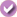Yes, I Did This Project! Please log in (or create a free account) to let us know how things went.

## Credits

Ben Finio, PhD, Science Buddies
• Xpult is a registered trademark of Peloton Systems LLC.
• Wiffle is a registered trademark of The Wiffle Ball, Inc.
• QuickTime is a registered trademark of Apple.
• Excel is a registered trademark of Microsoft Corporation.

General citation information is provided here. Be sure to check the formatting, including capitalization, for the method you are using and update your citation, as needed.

### MLA Style

Finio, Ben. "Launch Time: The Physics of Catapult Projectile Motion." Science Buddies, 21 Sep. 2018, https://www.sciencebuddies.org/science-fair-projects/project-ideas/Phys_p089/physics/physics-of-catapult-projectile-motion. Accessed 13 Nov. 2019.

### APA Style

Finio, B. (2018, September 21). Launch Time: The Physics of Catapult Projectile Motion. Retrieved from https://www.sciencebuddies.org/science-fair-projects/project-ideas/Phys_p089/physics/physics-of-catapult-projectile-motion

Last edit date: 2018-09-21

## IntroductionYou have probably seen figures in your physics textbook that show a catapult launching a projectile and then equations that calculate the resulting trajectory. Well, it is a lot more fun if you actually get to use a catapult instead of just doing the calculations! In this science project, you will use a catapult to launch ping-pong balls and use a video camera to film their trajectory, or path, as they fly through the air. You can also use physics to predict the trajectory of the ball, and then compare this predicted trajectory to the one you measure from video recordings. If the results match, you can conclude that your predictions and the assumptions you used to make them were valid under the circumstances of the test. If they don't match exactly, that's OK. There are many real-world factors that can be difficult to account for in predictions. Part of the scientific process involves figuring out what those factors are so you can make better predictions next time.

Predicting the trajectory of a ball launched from the catapult requires an understanding of two fundamental physics concepts: projectile motion and conservation of energy.

### Projectile Motion

Projectile motion refers to the method used for calculating the trajectory of a projectile (which can be pretty much any physical object — a rock, a ball, etc.) as it moves through the air.

Technical Note:

You may hear "projectile motion" used interchangeably with the word kinematics, depending on the textbook, website, or teacher. Kinematics (the study of motion) broadly includes things like pulleys, linkages, and gears. For this project, we are only concerned with projectiles.

Understanding projectile motion requires an understanding of position (the location of the ball, or projectile), velocity (how fast the ball is moving), and acceleration (how fast the velocity changes). A more advanced understanding of projectile motion involves calculus and factors like air resistance. For this project we will use simplified constant acceleration equations. These equations rely on the assumption that we can ignore air resistance — otherwise, the acceleration would change over time, and not be constant. (Note: Hover over the equations in this Introduction with your cursor to view enlarged formulas.) The four equations are:

Equation 1:

Equation 2:

Equation 3:

Equation 4:

In these equations, the subscripts i and f stand for initial and final, respectively. The variables d, v, and a stand for distance, usually expressed in meters (m); velocity, usually expressed in meters per second (m/s); and acceleration, usually expressed in meters per second squared (m/s²). The variable t stands for elapsed time, usually expressed in seconds (s).

Given certain information about an object at one time, these equations let you calculate what is happening at another time. For example, if you are running at an initial velocity of 3 m/s and accelerating at a rate of 1 m/s², you can use Equation 2 to calculate that your final velocity will be 5 m/s after a time (t = 2) seconds passed by:

However, these equations are only for one dimension, and the catapult-launched ball in this experiment is going to be moving in two dimensions — x and y. Fortunately, we can take the same set of equations and apply them to the x and y directions independently — motion in the "y" direction does not affect motion on the "x" direction, and vice versa. Figure 1 shows a diagram of the setup for this experiment.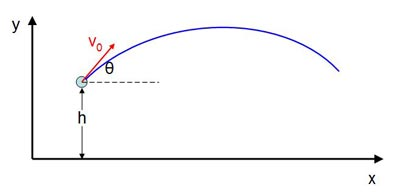Figure 1. This graphic shows a typical setup for a projectile motion problem. A projectile at height h above the ground (y=0) is launched with an initial velocity v0 at an angle θ with respect to the horizontal (the x-axis). Gravity (g) is in the negative y direction.

We want to calculate the position of the ball at any time, t. Thus we need equations for both x and y as functions of t. We will call these x(t) and y(t). Let us start with a new Equation 5 (using Equation 1), renaming the variables to be consistent with the x direction:

Equation 5:

Two things here can immediately make this equation easier to work with: we can assume that the initial x position is just zero, so x0=0. We also know that there is no acceleration in the x direction, because we are ignoring air resistance, and gravity certainly does not act sideways, so ax=0. Now, Equation 5 reduces to:

Equation 6:

But we were only giving the initial velocity of the ball, v0 , not the initial velocity in the x-direction, vx,0 . However, we know the launch angle, θ. So, we can use trigonometry to calculate the initial x velocity:

Equation 7:

Plug that into Equation 6, and we have our final equation for x as a function of time:

Equation 8:

That is only half of the problem, though. We need to do the same thing for the y direction. Let us take Equation 1 again, this time substituting the appropriate y variables:

Equation 9:

In this case, we cannot make any variables zero. We know from Figure 1 that the ball is launched from an initial height of h and that we have a downward (and therefore negative) acceleration of gravity, g. We can also calculate the initial velocity in the y direction, similar to what we did for the x direction in Equation 8, but this time using sine instead of cosine. Equation 9 thus becomes:

Equation 10:

Now we can use Equations 8 and 10 to plot the motion of a projectile in the (x,y) plane.

### Conservation of Energy

We are not finished yet, however — using Equations 8 and 10 requires that we know a few things — the height of the catapult above the ground, the angle at which the ball is launched, and its initial velocity. It is easy to measure the catapult's height with a tape measure, and the catapult in this kit makes it easy to measure the launch angle (more on that later). But what about the initial velocity of the ball? We cannot measure that directly, so we will use conservation of energy to estimate what it would be. In our case, we are dealing with kinetic energy (the energy an object with mass has according to its velocity) and potential energy (the energy that is stored in the rubber bands of the catapult). Note: We are ignoring a small amount of gravitational potential energy for now.

All types of energy are usually expressed in units of joules (or J). Conservation of energy states that, assuming losses like friction in a system are negligible, the sum of kinetic energy (or KE) and potential energy (or PE) always stays the same. This can be expressed in an equation:

Equation 11:

For this project, we will need to know the kinetic energy of a point mass (the ball or projectile can be approximated by a mass concentrated in a point), expressed as follows:

Equation 12:

and the kinetic energy of a rotating object:

Equation 13:

where m is the mass of an object (usually expressed in kilograms [kg]) and v its velocity; I the mass moment of inertia about the center of rotation (usually expressed in kilograms*meters squared [kg*m²]) and ω the angular velocity (expressed in radians per second [rad/s]).

We also need to determine the potential energy stored in a spring. Normally, for a linear spring, this would be expressed as:

Equation 14:

where k is the spring constant (usually expressed in newtons per meter, [N/m]) and Δx is the displacement from the spring's rest position (do not confuse this with the distance x in Figure 1—they are different things). However, the rubber bands that come with the catapult kit do not behave this way — they act as nonlinear springs. The equation for their potential energy as a function of displacement Δx is:

Equation 15:

This equation is specific to the rubber bands in this catapult kit and will not necessarily be true for every nonlinear spring. You can look in the Bibliography or any high-school physics textbook for more information on these topics. We have also included a Science Buddies reference page, Linear & Nonlinear Springs Tutorial if you would like to understand where Equations 14 and 15 come from.

### Applying These Principles to the Catapult

The catapult kit is pictured in Figure 2, with all of its parts labeled. This catapult has two key features: an adjustable pin lets you set the launch angle (the angle in which the ball travels relative to horizontal at launch, shown in Figure 1) and tick marks let you measure the pull-back angle (how far back the arm is pulled before launch). Also, Figure 3 shows a zoomed-in view of how to measure these angles and Figure 4 labels them for clarity. The catapult kit also comes with two different balls (ping-pong and Wiffle®) and enables you to adjust the number of rubber bands. See the Bibliography for more information about the catapult kit.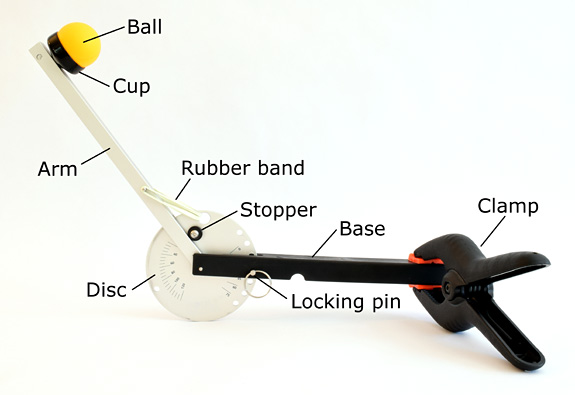Figure 2. The catapult with all of its parts labeled. The rubber-band-powered catapult is clamped to a table (we have used a paper towel to prevent it from scratching the surface). An aluminum disk with tick marks lets you measure the launch-angle and pull-back angle easily.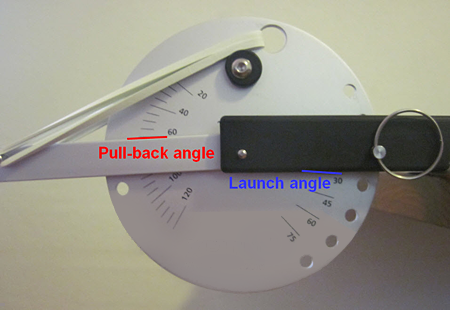Figure 3. Tick marks enable you to read the launch angle and the pull-back angle on the catapult. In this picture, the launch angle is 30 degrees and the pull-back angle is 60 degrees.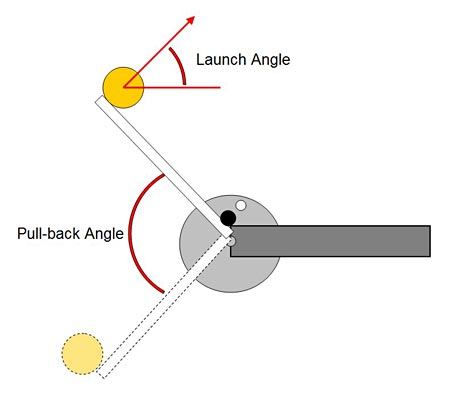Figure 4. Illustration of the launch angle and pull-back angle on the catapult.

Remember that our goal is to calculate the initial velocity of the ball, so we can then use the projectile motion equations to predict its path. To do this, we will compare the total energy of the catapult system in two different positions: when the arm is pulled back all the way, and immediately before the ball leaves the cup. These two positions are shown in Figure 5 with the following variables labeled:

• L is the length of the launch arm;
• r is the radius to the center of the ball (this is slightly smaller than L);
• x is the total length of the rubber band (it is folded in half for use on the catapult, so Figure 5 only shows ½ x); and
• v is the velocity of the ball.

Using Equations 11 through 14, we can write out the energy balance for these two positions. Three things can have energy in our system:

1. The ball can have kinetic energy (remember that we are ignoring gravity for now, since the elevation change is very small);
2. The arm of the catapult can have kinetic energy; and
3. The rubber bands can have potential energy.

The ball and the arm initially have zero velocity — and thus no kinetic energy. When you pull the arm back, potential energy is stored in the rubber bands. When you release the arm, the rubber bands contract, and their potential energy is converted to kinetic energy for the arm and the ball. The sum of these energies always has to be the same, which enables you to calculate the velocity of the ball. We will not go through the full derivation here — but advanced students may want to work this through and include it as part of their project. The energy balance, where N is the number of rubber bands, k is the spring constant of a single rubber band, x0 is the unstretched length of a rubber band, m the mass of the ball, and I is the moment of inertia of the arm, is:

Equation 16:

You will have to do some algebra to rearrange Equation 16 to solve for our unknown variable — the launch velocity of the ball, v2. Also note that this equation makes use of the relationship between the linear velocity of the ball, v, and the angular velocity of the arm, ω:

Equation 17:

Now that you know the launch velocity, you can go back to Equations 8 and 10, and you have everything you need to calculate the x and y positions of the ball at any time, t. This will give you your theoretical results or predicted trajectory — that is, what should happen according to equations you wrote down on paper. You will compare these to your experimental results — what actually happens when you launch the ball and measure its trajectory with recorded video.

A challenge for advanced students: The length of the rubber band, x, can just be measured with a ruler, but this could get annoying after a while. Can you use trigonometry to find a relationship between the length of the rubber band and the pull-back angle (which can be read directly from the disk on the catapult)?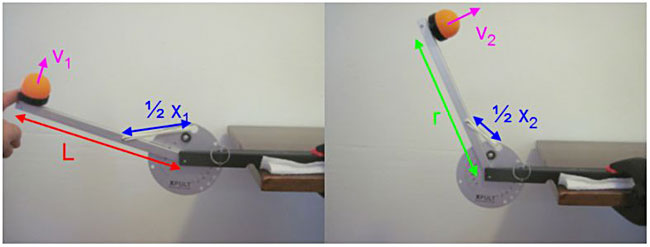Figure 5. The catapult when it is pulled back all the way (left), and immediately before the ball is launched (right). V is the velocity of the ball, x the total length of the rubber band, L the length of the launch arm, and r the radius to the center of the ball. L and r both remain constant, so we did not label them both in each half of the figure in order to avoid clutter; L goes all the way to the end of the launch arm while r's distance stops half way through the ball. Important: remember that the rubber band is folded in half when inserted into the catapult — thus, the side view shows only half of its total length.

## Terms and Concepts

You should be familiar with the following terms and concepts before continuing with the project. We have provided a brief explanation of projectile motion and conservation of energy above, but you may need to consult resources in the Bibliography if you do not understand them.

• Trajectory
• Kinematics
• Projectile motion
• Position
• Velocity
• Acceleration
• Constant acceleration equations
• Air resistance
• Conservation of Energy
• Kinetic Energy
• Potential Energy
• Spring constant
• Linear spring
• Nonlinear spring
• Launch angle
• Pull-back angle
• Theoretical results
• Experimental results

### Questions

• How well do you think your theoretical results (also called predictions) will agree with your experimental results? Several things could affect this:
• We ignored factors like gravitational potential energy and air resistance in the predictions. Do you think those will matter in the experiments, or will they be negligible?
• There are several physical constants in the equations, like the spring constant of the rubber band, the mass of the ping pong ball, and the moment of inertia of the launch arm. Do you think small errors in the values for these constants could have a big impact on the theoretical results?
• Your experimental results will depend on recorded videos of the ball's motion. Do you think you will be able to measure the ball's position in these videos accurately?
• If any of these things become problems, how could you address them to make your predictions or measurements more accurate?

## Bibliography

You can refer to the following references for more information about the physics principles involved in this project, as well as the original directions for the catapult kit from its manufacturer.

## News Feed on This Topic, ,
Note: A computerized matching algorithm suggests the above articles. It's not as smart as you are, and it may occasionally give humorous, ridiculous, or even annoying results! Learn more about the News Feed

## Materials and Equipment• Ping Pong Catapult Kit is available from our partner Home Science Tools. The kit includes:
• Three rubber bands, 3 × 1/8 inches
• Table tennis (ping pong) ball
• Plastic ball with holes (like a Wiffle® ball)
• Clamp for attaching the catapult to a surface
• Surface for mounting catapult (piece of wood on the floor, tabletop, etc.)
• Optional: paper towels, dish towel, or other padding for protecting the mounting surface from being scratched by the attachment clamp
• Open area for launching balls with bright lighting (either outside in direct sunlight, or inside with lots of lights)
• Video camera
• A higher quality camera will give better experimental results.
• A higher frame rate will also give better results (some cameras can film at 60 or 120 frames per second instead of just 30).
• The ability to manually adjust the camera's exposure time will help your videos be less blurry — the ball moves very fast!
• A tripod or other sturdy tool to mount and aim the camera is necessary — unless you have someone to help with a very steady hand!
• Ruler
• Media player program that enables you to step through videos frame-by-frame; Apple QuickTime® is a good option and can be downloaded for free.
• Microsoft Excel or other spreadsheet program
• Optional: a video tracking program like Tracker Video Analysis and Modeling, a free open-source video tracking program.
• Lab notebook

## Recommended Project SuppliesGet the right supplies — selected and tested to work with this project.
Project Kit: \$44.95

## Experimental Procedure

### Physical Constants

Before starting your experiments, you will need to measure or calculate several physical constants necessary for your theoretical results. You will need:

• The mass of the ping pong ball, m
• The radius from the center of rotation to the center of the ping pong ball, r
• The length of the launch arm, L
• The moment of inertia of the launch arm, I
• The unstretched length of a rubber band, x0
• The spring coefficient of a rubber band, k. (We use the word "coefficient" instead of "constant" since the rubber bands are nonlinear springs).
1. In case you do not have a scale, we will provide the mass of the ball for you (this was for the orange ping pong ball included with the catapult kit in September 2012; if this changes in the future, you will need to measure the mass of the new ball). We determined that m = 2.7 grams (g).
2. You should be able to measure the lengths L and r easily with a ruler; see Figure 5 in the Introduction.
3. The arm's moment of inertia I is a bit more complicated, as it cannot be measured directly with an instrument like a ruler or a scale. It must be calculated; we arrived at a value of I = 1.1 x 10-3kg*m².
Advanced students: Can you do this calculation yourself, and include it as part of your project? Assume the rod is made out of aluminum. Do different formulas for moment of inertia give you different results (for example, assuming the arm is a "thin rod", or a combination of rectangular prisms)?
4. For the rubber band, we recommend measuring your own values in case the type of rubber bands shipped with the catapult kit change over time. To measure x0, you will have to measure the length of the rubber band while it is unstretched but flat. Figure 6 shows how to do this.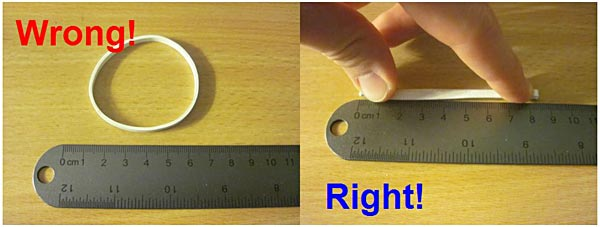Figure 6. The incorrect (left) and correct (right) ways to measure the unstretched length of the rubber band, x0. Note that in the image on the right, the rubber band is NOT being stretched — it is just pressed flat.
1. Measuring the spring coefficient k is slightly more complicated, because the rubber bands do not behave as linear springs. We found the value in Equation 15 (from the Introduction) to be k = 33.55 N/m½. (Note that for a linear spring, k always has units of N/m — in this case the units are different because the spring is nonlinear).
Advanced students: Can you calculate this value yourself and include it as part of your project?

### Theoretical Predictions

1. Select a launch angle, pull-back angle, and number of rubber bands that you would like to test.
1. Using Equations 8 and 10 from the Introduction, fill out a table or spreadsheet of the expected position of the ball at different times (remember that first you will have to use Equation 16 to calculate the launch velocity). We recommend starting at time t=0 and going up to t=1 second (the ball is usually in the air for less than a second, so this should give you more than enough theoretical data), in intervals of 1/30th of a second (this will correspond to the frame rate of most video cameras).
2. This will be easiest with a spreadsheet program such as Microsoft Excel®.
3. You can add your experimental results to this spreadsheet later, to make it easier to compare the two, as in Table 1.
Theoretical Experimental
Time
(seconds)
x
(meters)
y
(meters)
x
(meters)
y
(meters)
0.000
0.033
0.067
0.100
0.133
0.167
0.200
Table 1. A data table such as this enables you to gather theoretical predictions as well as experimental measurements and compare the two.

### Filming the Ball

1. Watch this video to learn how to set up your catapult:
Video instructions for using your ping pong catapult.
1. Next you will need to find an open, well-lit area to film a launch, using the same catapult settings you just used for your theoretical predictions. Make sure your camera is far enough away that you can see the catapult itself, and that the ball does not go off-screen before it hits the ground. If you can adjust settings on your camera, like the exposure time or frame rate, you may need to take several videos to find the best settings. Your goal is to be able to clearly see the location of the ball in each frame, without blurring. Important: You will need an object with a known length (such as a ruler) in the video for scale. This will enable you to convert the distance you measure on your computer screen to real-world distance.
2. Also remember to measure and record the length of the rubber band when the launch arm is pulled back to your desired pull-back angle, and at launch (when the arm is all the way up against the rubber stopper). Remember that you need to measure the total length of the rubber band, which is folded in half.
3. To make sure that your launch is not a fluke (which could really throw you off when you compare your experimental results to your theoretical data), we recommend filming at least three launches with the same settings.

1. Upload your videos to a computer, and open them in a program that can play them frame-by-frame (we recommend Apple QuickTime®, as other players often do not let you step through videos one frame at a time). Using a ruler, measure the x and y positions of the ball in each frame, starting from the moment the ball leaves the launch cup (be careful not to scratch your computer screen with the ruler). Remember that in the Introduction, we defined the ground as y=0, with the ball leaving the launch cup at height h above the ground, and the ball starting off at x=0.
• Optional: instead of measuring the ball's position on your screen with a ruler, you can open your video file in a program like Tracker Video Analysis and Modeling, which will allow you to click on the ball in each frame of your video to record its location. You will need to read the instructions for the Tracker program and learn how to use it.
2. Important: Remember that the distances you measure on your computer screen are not the same as the real-life position of the ball! You should have had a scale in your videos — we will use a 30-centimeter (cm) ruler as an example. Say a 30 cm ruler in your video appears to be 3 cm long on your computer screen. This means that your video has a scale of 30:3, or 10:1. So, to convert your on-screen measurements, to real-life values, you multiply all of them by 10. For example:
• You measure the (x,y) position of the ball on your computer screen to be x = 4 cm, y = 2 cm.
• Multiply each of these values by 10 to get the actual position of the ball: x = 40 cm, y = 20 cm.
• Remember that your video might have a different scale factor! This is just an example.
• The scale factor will change if you change the size of the window on your computer screen. If you open the program, calculate your scale factor, and then make the video full-screen, your scale factor will no longer be valid.

Enter your experimental results from your video analysis in the same table or spreadsheet as your theoretical results. Now you can plot the (x,y) position of the ball for both theoretical and experimental results on the same graph. Are they the same?

If your experimental and theoretical results are different, can you explain why? Can you do multiple trials with different launch settings to see if some match up better than others?

### Troubleshooting

For troubleshooting tips, please read our FAQ: Launch Time: The Physics of Catapult Projectile Motion.

## If you like this project, you might enjoy exploring these related careers:

### Mechanical Engineer

Mechanical engineers are part of your everyday life, designing the spoon you used to eat your breakfast, your breakfast's packaging, the flip-top cap on your toothpaste tube, the zipper on your jacket, the car, bike, or bus you took to school, the chair you sat in, the door handle you grasped and the hinges it opened on, and the ballpoint pen you used to take your test. Virtually every object that you see around you has passed through the hands of a mechanical engineer. Consequently, their skills are in demand to design millions of different products in almost every type of industry. Read more

### Physicist

Physicists have a big goal in mind—to understand the nature of the entire universe and everything in it! To reach that goal, they observe and measure natural events seen on Earth and in the universe, and then develop theories, using mathematics, to explain why those phenomena occur. Physicists take on the challenge of explaining events that happen on the grandest scale imaginable to those that happen at the level of the smallest atomic particles. Their theories are then applied to human-scale projects to bring people new technologies, like computers, lasers, and fusion energy. Read more

### Mechanical Engineering Technician

You use mechanical devices every day—to zip and snap your clothing, open doors, refrigerate and cook your food, get clean water, heat your home, play music, surf the Internet, travel around, and even to brush your teeth. Virtually every object that you see around has been mechanically engineered or designed at some point, requiring the skills of mechanical engineering technicians to create drawings of the product, or to build and test models of the product to find the best design. Read more

### Mathematician

Mathematicians are part of an ancient tradition of searching for patterns, conjecturing, and figuring out truths based on rigorous deduction. Some mathematicians focus on purely theoretical problems, with no obvious or immediate applications, except to advance our understanding of mathematics, while others focus on applied mathematics, where they try to solve problems in economics, business, science, physics, or engineering. Read more

## Variations

• The theoretical predictions outlined in this project ignore gravitational potential energy during launch and air resistance during the ball's flight. Can you re-derive the energy and projectile motion equations to include these terms? If so, do the theoretical results match up better with your experiments?
• Are your results different if you use the Wiffle® ball instead of the ping pong ball?

## Share your story with Science Buddies!Yes, I Did This Project! Please log in (or create a free account) to let us know how things went.

The Science Buddies catapult kit is used in four projects:

The FAQs below are used for all four projects. General catapult questions are at the top of the FAQs list and project specific questions are at the bottom of the list.

Q: The ball doesn't travel exactly the same distance each time, even when I use the exact same settings. What am I doing wrong?
A: This is OK! Due to many small, random effects beyond your control, like the catapult wiggling a bit between shots, or even air currents in the room, the ball will not travel exactly the same distance every time. In almost all "real world" experiments, you will not get the exact same results every single time.
Q: My catapult seems to move side-to-side slightly after each shot. How can I prevent this?
A: Depending on the surface material you are clamping to, the single clamp provided with the catapult kit may not hold the catapult perfectly still, especially when using two or three rubber bands. The catapult may wiggle a bit after each shot. This wiggle will may affect your left-to-right aim but will not have a significant effect on your distance or vertical trajectory. If you would like to eliminate the wiggle try stacking heavy objects, like textbooks, on top of the catapult base — just make sure they don't interfere with movement of the launch arm.
Q: How do I set the launch angle on the catapult?
A: The launch angle is set by inserting a metal pin through holes on the aluminum disk that align with the black metal base of the catapult. This picture shows the process: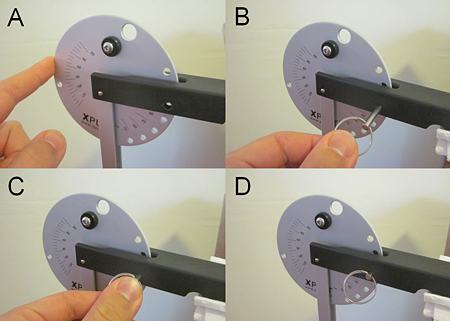Q: How do I attach the rubber bands to the catapult?
A: The rubber bands go through a hole in the circular aluminum disk, and hook onto pins on the launch arm. This picture shows the process in four steps:Q: How do I measure the launch angle/pull-back angle?
A: The launch angle and pull-back angle are measured by tick marks on the side of the aluminum disk. When facing the writing on the side of the catapult, the launch angle tick marks will be on your right — they line up with the bottom edge of the base of the catapult (the black part that should be clamped to a table). The pull-back angle tick marks are on your left — they line up with the top edge of the launch arm. This picture shows how to read each angle:Note: On older versions of the Xpult brand catapult, the pull-back angle may be read from the bottom edge of the launch arm. If you just ordered a new Science Buddies catapult kit for your project (as of October 2012), then you should follow the directions above.

Q: How can I stop the ball from bouncing out of the target in the Bombs Away! A Ping Pong Catapult project?
A: The ball is likely to bounce out of targets like metal pots or hard plastic trash cans, which can make chasing it all over the place annoying (especially if it rolls under furniture). Place a crumpled-up hand towel or t-shirt in the bottom of your target to reduce bouncing. Remember though - any shot that lands inside the target, even if it bounces out, still counts as a successful hit.
Q: I'm having trouble knocking over the box wall in the Under Siege! Use a Catapult to Storm Castle Walls project. What should I do?
A: First, make sure the box you are using for a castle wall isn't too big or heavy. We found that empty cereal boxes work well. A full cereal box will be much too heavy. Second, adjust your settings and aim — try using a different number of rubber bands, or switching your aim to a different part of the wall (top/bottom/middle). Remember, change one variable at a time and keep a good data table in order to figure out what is working!
Q: I'm having trouble filming the ball in Launch Time: The Physics of Catapult Projectile Motion project — it always looks blurry in each frame of my video. What can I do?
A: This is most likely due to poor lighting conditions. Many video cameras will automatically adjust their exposure time depending on the brightness of the scene. A poorly lit area will result in a longer exposure time, to let more light into the camera — however, this will also make fast-moving things appear blurry. If possible, try filming outside in direct sunlight (but not on a windy day!), or use extra lamps if filming indoors (remove lampshades to increase brightness).

Depending on your camera, you may also be able to manually adjust settings and decrease the exposure time. Consult your camera's manual to see if that is possible.

Q: My experimental results do not match up with my theoretical predictions in Launch Time: The Physics of Catapult Projectile Motion project. Am I doing something wrong?
A: If your theoretical and experimental results don't match up exactly, but are "reasonably" close to each other (for example, both show the ball going a couple meters before it hits the ground), then this is perfectly normal. There are parameters in both the theoretical predictions and the experiments that can vary slightly and affect your results. For example, the spring coefficient of your rubber bands could be slightly different than the number we provided, and if your catapult is not clamped down very well, the actual launch angle could be slightly different from the launch angle you set, as the catapult can "bounce" a bit during launch. All of these factors can contribute to make your experimental and theoretical results slightly different — but this is OK.

If your theoretical and experimental results are very different, there are a couple things you can check:

• Are your theoretical predictions reasonable? You should be able to launch the ball across an average-sized room in your house — meaning it should go a couple meters before it hits the ground, for typical catapult settings. If your predictions indicate that the ball will travel several kilometers, or only a couple millimeters, then odds are you made a mathematical mistake. Double-check your calculations, and be extra careful to look for typos if you entered equations into a spreadsheet program.
• Make sure you properly used a scale factor to convert the distances you measure on your computer screen to real-world distances for your experimental data. Again, make sure the distance the ball travels is reasonable — if your experimental data says that the ball traveled 100 meters, then you probably used the scale factor incorrectly.
Q: I can't get a nice histogram for the Bet You Can't Hit Me! The Science of Catapult Statistics project. What am I doing wrong?
A: The first thing you can do is try to adjust the bin size of your histogram — how you group and count the frequency of your data. A bin size that is too small or too large will make it difficult to see the true shape of the distribution. Here are four different histograms for the same set of data, all with different bin sizes: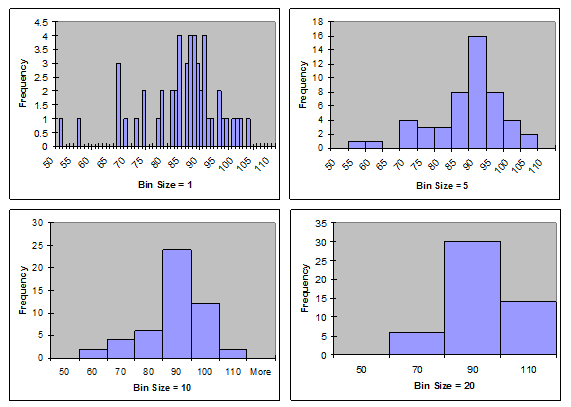Notice how the shape of the distribution is evident with bin sizes of 5 or 10, but rather difficult to see with bin sizes of 1 or 20. As a general rule of thumb, more data will allow you to use a smaller bin size, which will give you a more accurate picture of the distribution — so if you have time, try to do more than 50 trials with the catapult.

Q: I'm having trouble accurately recording the distance the ball travels in the Bet You Can't Hit Me! The Science of Catapult Statistics project. What am I doing wrong?
A: First, it is essential to have two people for this part of the project — one to operate the Xpult, and another to record the distance the ball travels. You won't be able to do both by yourself.

For the person recording distances, we recommend watching where the ball lands and immediately marking the location with your finger. Then take a reading from the tape measure. Trying to watch where the ball lands and simultaneously read the tape measure will be very difficult.

Also, you probably won't be able to get fraction-of-an-inch accuracy on your readings — getting the closest one-inch increment will be good enough.

The Ask an Expert Forum is intended to be a place where students can go to find answers to science questions that they have been unable to find using other resources. If you have specific questions about your science fair project or science fair, our team of volunteer scientists can help. Our Experts won't do the work for you, but they will make suggestions, offer guidance, and help you troubleshoot.

If you have purchased a kit for this project from Science Buddies, we are pleased to answer any question not addressed by the FAQ above.

1. What is your Science Buddies kit order number?
2. Please describe how you need help as thoroughly as possible:

Examples

Good Question I'm trying to do Experimental Procedure step #5, "Scrape the insulation from the wire. . ." How do I know when I've scraped enough?
Good Question I'm at Experimental Procedure step #7, "Move the magnet back and forth . . ." and the LED is not lighting up.
Bad Question I don't understand the instructions. Help!
Good Question I am purchasing my materials. Can I substitute a 1N34 diode for the 1N25 diode called for in the material list?
Bad Question Can I use a different part?